Batch Normalization.¶

Batch normalization has become one important operation in faster and stable learning of neural networks. In batch norm we do the following: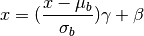The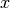is the input (and the output) of this operation,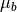and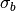are the mean and the variance of the minibatch ofsupplied.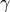and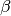are learnt using back propagation. This will also store a running mean and a running variance, which is used during inference time.

By default batch normalization can be performed on convolution and dot product layers using the argument batch_norm = True supplied to the yann.network.add_layer method. This will apply the batch normalization before the activation and after the core layer operation.

While this is the technique that was described in the original batch normalization paper. Some modern networks such as the Residual network , use a re-orderd version of layer operations that require the batch norm to be applied post-activation. This is particularly used when using ReLU or Maxout networks. Therefore we also provide a layer type batch_norm, that could create a layer that simply does batch normalization on the input supplied. These layers could be used to create a post-activation batch normalization.

This tutorial demonstrates the use of both these techniques using the same architecutre of networks used in the Convolutional Neural Network. tutorial. The codes for these can be found in the following module methods in pantry.tutorials.

References

  Ioffe, Sergey, and Christian Szegedy. “Batch normalization: Accelerating deep network training by reducing internal covariate shift.” arXiv preprint arXiv:1502.03167 (2015).
  He, Kaiming, et al. “Identity mappings in deep residual networks.” European Conference on Computer Vision. Springer International Publishing, 2016.
  He, Kaiming, et al. “Deep residual learning for image recognition.” Proceedings of the IEEE Conference on Computer Vision and Pattern Recognition. 2016.
  Nair, Vinod, and Geoffrey E. Hinton. “Rectified linear units improve restricted boltzmann machines.” Proceedings of the 27th International Conference on Machine Learning (ICML-10). 2010.
  Goodfellow, Ian J., et al. “Maxout networks.” arXiv preprint arXiv:1302.4389 (2013).

Notes

This code contains three methods.
1. A modern reincarnation of LeNet5 for MNIST.
2. The same Lenet with batchnorms
2.a. Batchnorm before activations. 2.b. Batchnorm after activations.

All these methods are setup for MNIST dataset.

Todo

pantry.tutorials.lenet.lenet5(dataset=None, verbose=1)[source]

This function is a demo example of lenet5 from the infamous paper by Yann LeCun. This is an example code. You should study this code rather than merely run it.

Warning

This is not the exact implementation but a modern re-incarnation.

Parameters: dataset – Supply a dataset. verbose – Similar to the rest of the dataset.
pantry.tutorials.lenet.lenet_maxout_batchnorm_after_activation(dataset=None, verbose=1)[source]

This is a version with nesterov momentum and rmsprop instead of the typical sgd. This also has maxout activations for convolutional layers, dropouts on the last convolutional layer and the other dropout layers and this also applies batch norm to all the layers. The difference though is that we use the batch_norm layer to apply batch norm that applies batch norm after the activation fo the previous layer. So we just spice things up and add a bit of steroids to lenet5(). This also introduces a visualizer module usage.

Parameters: dataset – Supply a dataset. verbose – Similar to the rest of the dataset.
pantry.tutorials.lenet.lenet_maxout_batchnorm_before_activation(dataset=None, verbose=1)[source]

This is a version with nesterov momentum and rmsprop instead of the typical sgd. This also has maxout activations for convolutional layers, dropouts on the last convolutional layer and the other dropout layers and this also applies batch norm to all the layers. The batch norm is applied by using the batch_norm = True parameters in all layers. This batch norm is applied before activation as is used in the original version of the paper. So we just spice things up and add a bit of steroids to lenet5(). This also introduces a visualizer module usage.

Parameters: dataset – Supply a dataset. verbose – Similar to the rest of the dataset.